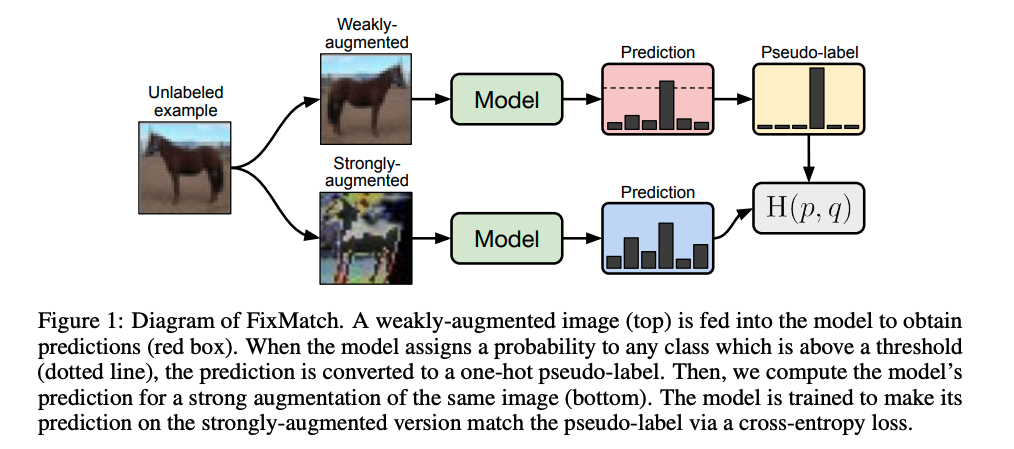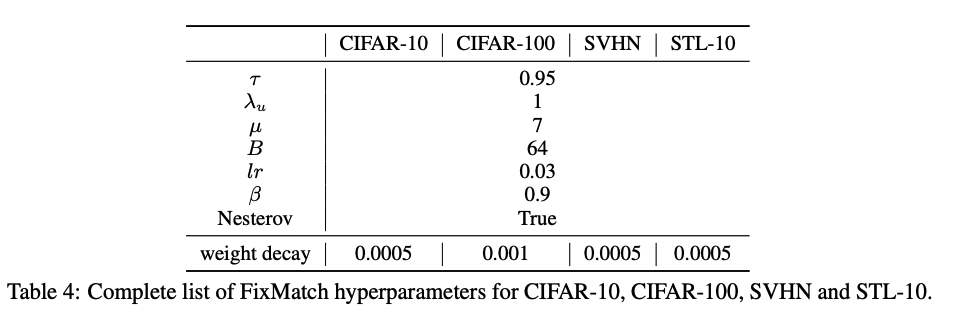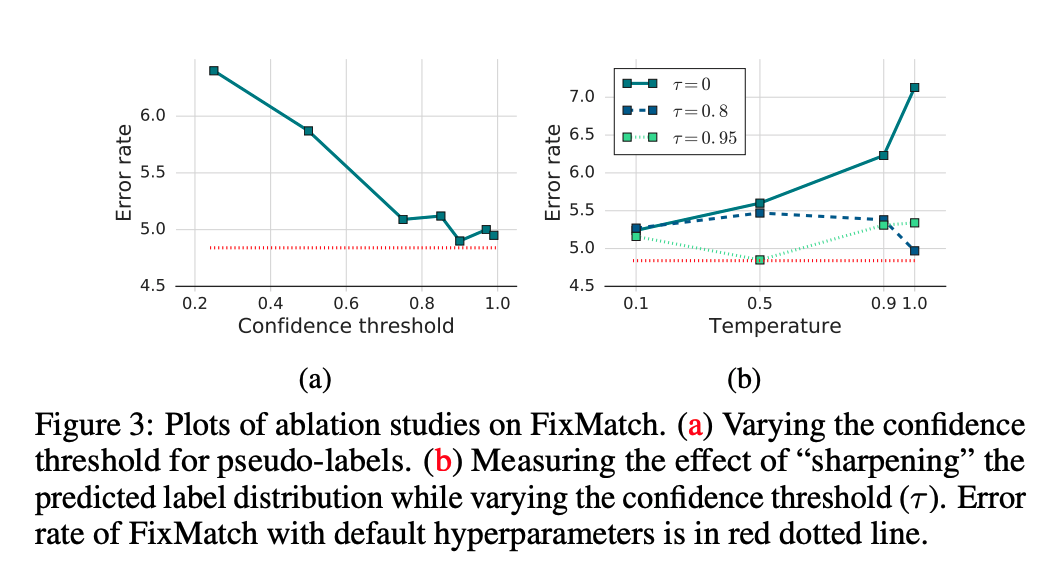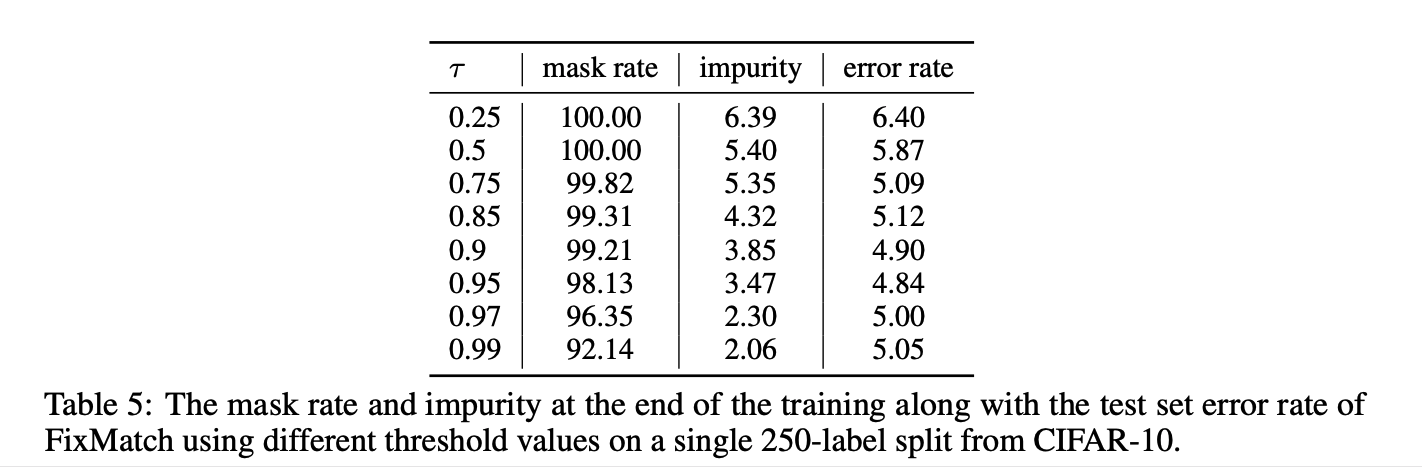## 方法FixMatch训练过程包含两部分：有监督训练和无监督训练。对于有标注数据，进行常规的有监督训练过程即可，这里我们主要重点介绍无监督训练过程，从上图可以看到，对于每张未标注的图像，使用弱增强和强增强获得两张增强图像。首先，弱增强图像通过模型得到预测得分，当模型的预测得分高于一定的阈值时，对应的类别作为 ground truth 的标签，即伪标签。然后，强增强的图像通过相同模型得到预测得分，并使用交叉熵损失将此预测得分与 ground truth 伪标签进行比较。

$\ell_{s}=\frac{1}{B} \sum_{b=1}^{B} \mathrm{H}\left(p_{b}, p_{\mathrm{m}}\left(y \mid \alpha\left(x_{b}\right)\right)\right)$

$\ell_{u}=\frac{1}{\mu B} \sum_{b=1}^{\mu B} \mathbb{1}\left(\max \left(q_{b}\right) \geq \tau\right) \mathrm{H}\left(\hat{q}_{b}, p_{\mathrm{m}}\left(y \mid \mathcal{A}\left(u_{b}\right)\right)\right)$

$\ell_{s}+\lambda_{u} \ell_{u}$

## 实验

### 实验参数### 实验结果1. FixMatch 在 CIFAR-10 和 SVHN 基准测试中获得了 state of the art 的结果。

2. 在 CIFAR-100 上，ReMixMatch 优于 FixMatch。为了理解原因，作者从 ReMixMatch 中借用了各种组件到 FixMatch 上，并测量了它们对性能的影响。他们发现，*Distribution Alignment（DA)*组件促使模型以相同的概率预测所有类，这就是原因。因此，当他们将 FixMatch 与 DA 结合使用时，他们实现了 40.14％的错误率，而 ReMixMatch 的错误率为 44.28％。

3. STL-10 数据集由 100,000 个未标注图像和 5000 个标注图像组成。我们需要预测 10 类（飞机，鸟，汽车，猫，鹿，狗，马，猴子，船，卡车）。它是半监督学习的更具代表性的评估方法，因为其未标注的集合具有分布以外的图像。在所有方法中，对 1000 张带标签的图像进行 5 折评估时，FixMatch 的 CTAugment 可以实现最低的错误率。